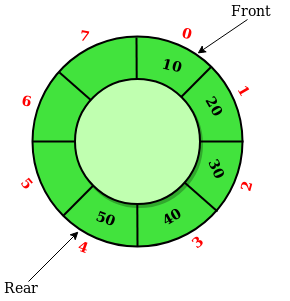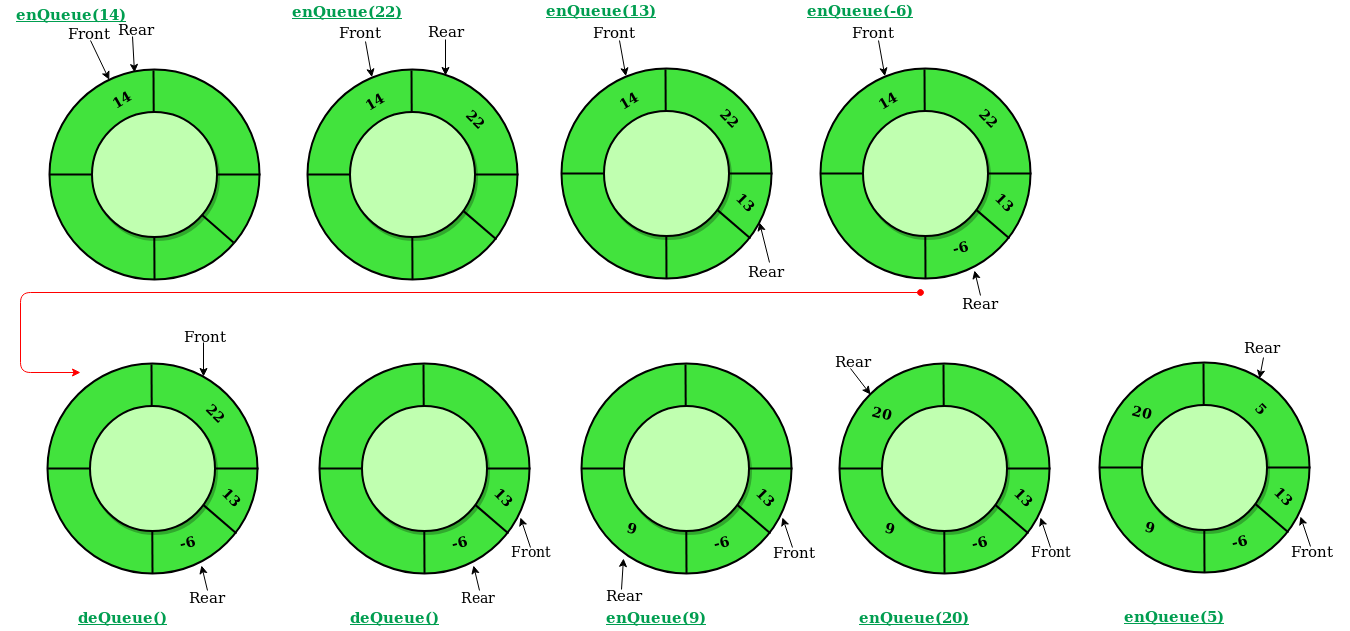# Circular Queue | Set 1 (Introduction and Array Implementation)

Prerequisite – Queues
Circular Queue is a linear data structure in which the operations are performed based on FIFO (First In First Out) principle and the last position is connected back to the first position to make a circle. It is also called ‘Ring Buffer’In a normal Queue, we can insert elements until queue becomes full. But once queue becomes full, we can not insert the next element even if there is a space in front of queue.Operations on Circular Queue:

• Front: Get the front item from queue.
• Rear: Get the last item from queue.
• enQueue(value) This function is used to insert an element into the circular queue. In a circular queue, the new element is always inserted at Rear position.
Steps:

1. Check whether queue is Full – Check ((rear == SIZE-1 && front == 0) || (rear == front-1)).
2. If it is full then display Queue is full. If queue is not full then, check if (rear == SIZE – 1 && front != 0) if it is true then set rear=0 and insert element.
• deQueue() This function is used to delete an element from the circular queue. In a circular queue, the element is always deleted from front position.
Steps:

1. Check whether queue is Empty means check (front==-1).
2. If it is empty then display Queue is empty. If queue is not empty then step 3
3. Check if (front==rear) if it is true then set front=rear= -1 else check if (front==size-1), if it is true then set front=0 and return the element.

## C++

 `// C or C++ program for insertion and ` `// deletion in Circular Queue ` `#include ` `using` `namespace` `std; ` ` `  `struct` `Queue ` `{ ` `    ``// Initialize front and rear ` `    ``int` `rear, front; ` ` `  `    ``// Circular Queue ` `    ``int` `size; ` `    ``int` `*arr; ` ` `  `    ``Queue(``int` `s) ` `    ``{ ` `       ``front = rear = -1; ` `       ``size = s; ` `       ``arr = ``new` `int``[s]; ` `    ``} ` ` `  `    ``void` `enQueue(``int` `value); ` `    ``int` `deQueue(); ` `    ``void` `displayQueue(); ` `}; ` ` `  ` `  `/* Function to create Circular queue */` `void` `Queue::enQueue(``int` `value) ` `{ ` `    ``if` `((front == 0 && rear == size-1) || ` `            ``(rear == (front-1)%(size-1))) ` `    ``{ ` `        ``printf``(``"\nQueue is Full"``); ` `        ``return``; ` `    ``} ` ` `  `    ``else` `if` `(front == -1) ``/* Insert First Element */` `    ``{ ` `        ``front = rear = 0; ` `        ``arr[rear] = value; ` `    ``} ` ` `  `    ``else` `if` `(rear == size-1 && front != 0) ` `    ``{ ` `        ``rear = 0; ` `        ``arr[rear] = value; ` `    ``} ` ` `  `    ``else` `    ``{ ` `        ``rear++; ` `        ``arr[rear] = value; ` `    ``} ` `} ` ` `  `// Function to delete element from Circular Queue ` `int` `Queue::deQueue() ` `{ ` `    ``if` `(front == -1) ` `    ``{ ` `        ``printf``(``"\nQueue is Empty"``); ` `        ``return` `INT_MIN; ` `    ``} ` ` `  `    ``int` `data = arr[front]; ` `    ``arr[front] = -1; ` `    ``if` `(front == rear) ` `    ``{ ` `        ``front = -1; ` `        ``rear = -1; ` `    ``} ` `    ``else` `if` `(front == size-1) ` `        ``front = 0; ` `    ``else` `        ``front++; ` ` `  `    ``return` `data; ` `} ` ` `  `// Function displaying the elements ` `// of Circular Queue ` `void` `Queue::displayQueue() ` `{ ` `    ``if` `(front == -1) ` `    ``{ ` `        ``printf``(``"\nQueue is Empty"``); ` `        ``return``; ` `    ``} ` `    ``printf``(``"\nElements in Circular Queue are: "``); ` `    ``if` `(rear >= front) ` `    ``{ ` `        ``for` `(``int` `i = front; i <= rear; i++) ` `            ``printf``(``"%d "``,arr[i]); ` `    ``} ` `    ``else` `    ``{ ` `        ``for` `(``int` `i = front; i < size; i++) ` `            ``printf``(``"%d "``, arr[i]); ` ` `  `        ``for` `(``int` `i = 0; i <= rear; i++) ` `            ``printf``(``"%d "``, arr[i]); ` `    ``} ` `} ` ` `  `/* Driver of the program */` `int` `main() ` `{ ` `    ``Queue q(5); ` ` `  `    ``// Inserting elements in Circular Queue ` `    ``q.enQueue(14); ` `    ``q.enQueue(22); ` `    ``q.enQueue(13); ` `    ``q.enQueue(-6); ` ` `  `    ``// Display elements present in Circular Queue ` `    ``q.displayQueue(); ` ` `  `    ``// Deleting elements from Circular Queue ` `    ``printf``(``"\nDeleted value = %d"``, q.deQueue()); ` `    ``printf``(``"\nDeleted value = %d"``, q.deQueue()); ` ` `  `    ``q.displayQueue(); ` ` `  `    ``q.enQueue(9); ` `    ``q.enQueue(20); ` `    ``q.enQueue(5); ` ` `  `    ``q.displayQueue(); ` ` `  `    ``q.enQueue(20); ` `    ``return` `0; ` `} `

## Java

 `// Java program for insertion and ` `// deletion in Circular Queue ` `import` `java.util.ArrayList; ` ` `  `class` `CircularQueue{ ` ` `  `// Declaring the class variables. ` `private` `int` `size, front, rear; ` ` `  `// Declaring array list of integer type. ` `private` `ArrayList queue = ``new` `ArrayList(); ` ` `  `// Constructor ` `CircularQueue(``int` `size) ` `{ ` `    ``this``.size = size; ` `    ``this``.front = ``this``.rear = -``1``; ` `} ` ` `  `// Method to insert a new element in the queue. ` `public` `void` `enQueue(``int` `data) ` `{ ` `     `  `    ``// Condition if queue is full. ` `    ``if``((front == ``0` `&& rear == size - ``1``) || ` `      ``(rear == (front - ``1``) % (size - ``1``))) ` `    ``{ ` `        ``System.out.print(``"Queue is Full"``); ` `    ``} ` ` `  `    ``// condition for empty queue. ` `    ``else` `if``(front == -``1``) ` `    ``{ ` `        ``front = ``0``; ` `        ``rear = ``0``; ` `        ``queue.add(rear, data); ` `    ``} ` ` `  `    ``else` `if``(rear == size - ``1` `&& front != ``0``) ` `    ``{ ` `        ``rear = ``0``; ` `        ``queue.set(rear, data); ` `    ``} ` ` `  `    ``else` `    ``{ ` `        ``rear = (rear + ``1``); ` `     `  `        ``// Adding a new element if  ` `        ``if``(front <= rear) ` `        ``{ ` `            ``queue.add(rear, data); ` `        ``} ` `     `  `        ``// Else updating old value ` `        ``else` `        ``{ ` `            ``queue.set(rear, data); ` `        ``} ` `    ``} ` `} ` ` `  `// Function to dequeue an element ` `// form th queue. ` `public` `int` `deQueue() ` `{ ` `    ``int` `temp; ` ` `  `    ``// Condition for empty queue. ` `    ``if``(front == -``1``) ` `    ``{ ` `        ``System.out.print(``"Queue is Empty"``); ` `         `  `        ``// Return -1 in case of empty queue ` `        ``return` `-``1``;  ` `    ``} ` ` `  `    ``temp = queue.get(front); ` ` `  `    ``// Condition for only one element ` `    ``if``(front == rear) ` `    ``{ ` `        ``front = -``1``; ` `        ``rear = -``1``; ` `    ``} ` ` `  `    ``else` `if``(front == size - ``1``) ` `    ``{ ` `        ``front = ``0``; ` `    ``} ` `    ``else` `    ``{ ` `        ``front = front + ``1``; ` `    ``} ` `     `  `    ``// Returns the dequeued element ` `    ``return` `temp; ` `} ` ` `  `// Method to display the elements of queue ` `public` `void` `displayQueue() ` `{ ` `     `  `    ``// Condition for empty queue. ` `    ``if``(front == -``1``) ` `    ``{ ` `        ``System.out.print(``"Queue is Empty"``); ` `        ``return``; ` `    ``} ` ` `  `    ``// If rear has not crossed the max size ` `    ``// or queue rear is still greater then ` `    ``// front. ` `    ``System.out.print(``"Elements in the "` `+ ` `                     ``"circular queue are: "``); ` ` `  `    ``if``(rear >= front) ` `    ``{ ` `     `  `        ``// Loop to print elements from ` `        ``// front to rear. ` `        ``for``(``int` `i = front; i <= rear; i++) ` `        ``{ ` `            ``System.out.print(queue.get(i)); ` `            ``System.out.print(``" "``); ` `        ``} ` `        ``System.out.println(); ` `    ``} ` ` `  `    ``// If rear crossed the max index and ` `    ``// indexing has started in loop ` `    ``else` `    ``{ ` `         `  `        ``// Loop for printing elements from ` `        ``// front to max size or last index ` `        ``for``(``int` `i = front; i < size; i++) ` `        ``{ ` `            ``System.out.print(queue.get(i)); ` `            ``System.out.print(``" "``); ` `        ``} ` ` `  `        ``// Loop for printing elements from ` `        ``// 0th index till rear position ` `        ``for``(``int` `i = ``0``; i <= rear; i++) ` `        ``{ ` `            ``System.out.print(queue.get(i)); ` `            ``System.out.print(``" "``); ` `        ``} ` `        ``System.out.println(); ` `    ``} ` `} ` ` `  `// Driver code ` `public` `static` `void` `main(String[] args) ` `{ ` `     `  `    ``// Initialising new object of ` `    ``// CircularQueue class. ` `    ``CircularQueue q = ``new` `CircularQueue(``5``); ` `     `  `    ``q.enQueue(``14``); ` `    ``q.enQueue(``22``); ` `    ``q.enQueue(``13``); ` `    ``q.enQueue(-``6``); ` `     `  `    ``q.displayQueue(); ` ` `  `    ``int` `x = q.deQueue(); ` ` `  `    ``// Checking for empty queue. ` `    ``if``(x != -``1``) ` `    ``{ ` `        ``System.out.print(``"Deleted value = "``); ` `        ``System.out.println(x); ` `    ``} ` ` `  `    ``x = q.deQueue(); ` ` `  `    ``// Checking for empty queue. ` `    ``if``(x != -``1``) ` `    ``{ ` `        ``System.out.print(``"Deleted value = "``); ` `        ``System.out.println(x); ` `    ``} ` ` `  `    ``q.displayQueue(); ` `     `  `    ``q.enQueue(``9``); ` `    ``q.enQueue(``20``); ` `    ``q.enQueue(``5``); ` `     `  `    ``q.displayQueue(); ` `     `  `    ``q.enQueue(``20``); ` `} ` `} ` ` `  `// This code is contributed by Amit Mangal. `

## Python 3

 `class` `CircularQueue(): ` ` `  `    ``# constructor ` `    ``def` `__init__(``self``, size): ``# initializing the class ` `        ``self``.size ``=` `size ` `         `  `        ``# initializing queue with none ` `        ``self``.queue ``=` `[``None` `for` `i ``in` `range``(size)]  ` `        ``self``.front ``=` `self``.rear ``=` `-``1` ` `  `    ``def` `enqueue(``self``, data): ` `         `  `        ``# condition if queue is full ` `        ``if` `((``self``.rear ``+` `1``) ``%` `self``.size ``=``=` `self``.front):  ` `            ``print``(``" Queue is Full\n"``) ` `             `  `        ``# condition for empty queue ` `        ``elif` `(``self``.front ``=``=` `-``1``):  ` `            ``self``.front ``=` `0` `            ``self``.rear ``=` `0` `            ``self``.queue[``self``.rear] ``=` `data ` `        ``else``: ` `             `  `            ``# next position of rear ` `            ``self``.rear ``=` `(``self``.rear ``+` `1``) ``%` `self``.size  ` `            ``self``.queue[``self``.rear] ``=` `data ` `             `  `    ``def` `dequeue(``self``): ` `        ``if` `(``self``.front ``=``=` `-``1``): ``# codition for empty queue ` `            ``print` `(``"Queue is Empty\n"``) ` `             `  `        ``# condition for only one element ` `        ``elif` `(``self``.front ``=``=` `self``.rear):  ` `            ``temp``=``self``.queue[``self``.front] ` `            ``self``.front ``=` `-``1` `            ``self``.rear ``=` `-``1` `            ``return` `temp ` `        ``else``: ` `            ``temp ``=` `self``.queue[``self``.front] ` `            ``self``.front ``=` `(``self``.front ``+` `1``) ``%` `self``.size ` `            ``return` `temp ` ` `  `    ``def` `display(``self``): ` `     `  `        ``# condition for empty queue ` `        ``if``(``self``.front ``=``=` `-``1``):  ` `            ``print` `(``"Queue is Empty"``) ` ` `  `        ``elif` `(``self``.rear >``=` `self``.front): ` `            ``print``(``"Elements in the circular queue are:"``,  ` `                                              ``end ``=` `" "``) ` `            ``for` `i ``in` `range``(``self``.front, ``self``.rear ``+` `1``): ` `                ``print``(``self``.queue[i], end ``=` `" "``) ` `            ``print` `() ` ` `  `        ``else``: ` `            ``print` `(``"Elements in Circular Queue are:"``,  ` `                                           ``end ``=` `" "``) ` `            ``for` `i ``in` `range``(``self``.front, ``self``.size): ` `                ``print``(``self``.queue[i], end ``=` `" "``) ` `            ``for` `i ``in` `range``(``0``, ``self``.rear ``+` `1``): ` `                ``print``(``self``.queue[i], end ``=` `" "``) ` `            ``print` `() ` ` `  `        ``if` `((``self``.rear ``+` `1``) ``%` `self``.size ``=``=` `self``.front): ` `            ``print``(``"Queue is Full"``) ` ` `  `# Driver Code ` `ob ``=` `CircularQueue(``5``) ` `ob.enqueue(``14``) ` `ob.enqueue(``22``) ` `ob.enqueue(``13``) ` `ob.enqueue(``-``6``) ` `ob.display() ` `print` `(``"Deleted value = "``, ob.dequeue()) ` `print` `(``"Deleted value = "``, ob.dequeue()) ` `ob.display() ` `ob.enqueue(``9``) ` `ob.enqueue(``20``) ` `ob.enqueue(``5``) ` `ob.display() ` ` `  `# This code is contributed by AshwinGoel  `

Output:

```Elements in Circular Queue are: 14 22 13 -6
Deleted value = 14
Deleted value = 22
Elements in Circular Queue are: 13 -6
Elements in Circular Queue are: 13 -6 9 20 5
Queue is Full
```

Time Complexity: Time complexity of enQueue(), deQueue() operation is O(1) as there is no loop in any of the operation.
Applications:

1. Memory Management: The unused memory locations in the case of ordinary queues can be utilized in circular queues.
2. Traffic system: In computer controlled traffic system, circular queues are used to switch on the traffic lights one by one repeatedly as per the time set.
3. CPU Scheduling: Operating systems often maintain a queue of processes that are ready to execute or that are waiting for a particular event to occur.

Attention reader! Don’t stop learning now. Get hold of all the important DSA concepts with the DSA Self Paced Course at a student-friendly price and become industry ready.

My Personal Notes arrow_drop_up

Article Tags :
Practice Tags :

38

Please write to us at contribute@geeksforgeeks.org to report any issue with the above content.